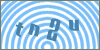Products
ServicesScripts: Javascript :: Date and Time Functions :: Library Article #26Developer's SectionAdding and Subtracting days to a Date Object in Javascript
By: Erobo Team Member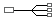Hire a Developer for Related Work / Installation | \$55 hr
 Rating: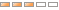| Rate It: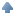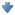Average Votes: (3002) Favorites:Learn a prototype method that can be used to create new date objects by adding / subtracting days to an existing date object.

In this tutorial you will learn a new Javascript prototype method that adds or subtracts days to a date object. This method will take as input a positive or negative integer number. When adding days you must enter a positive integer number, and a negative integer number when going back into the past. The object returned by this method can then be used to get the new resulting date object.

Example:

Add or subtract days to the date above:

The resulting date is:

Now Let's take a look at the Code Step by Step:

Create the prototype Date method: addDays(int value)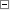Code Snippet 1<script language="javascript">
{

var targetDays = parseInt(s)
var thisYear = parseInt(this.getFullYear())
var thisDays = parseInt(this.getDate())
var thisMonth = parseInt(this.getMonth() + 1)

var currDays = thisDays;
var currMonth = thisMonth;
var currYear = thisYear;

var monthArr;

var nonleap = [31, 28, 31, 30, 31, 30, 31, 31, 30, 31, 30, 31];
// leap year
var leap = [31, 29, 31, 30, 31, 30, 31, 31, 30, 31, 30, 31];

if ((thisYear % 4) == 0) {
if ((thisYear % 100) == 0 && (thisYear % 400) != 0) { monthArr = nonleap; }
else { monthArr = leap; }
}
else {  monthArr = nonleap; }

var daysCounter = 0;
var numDays = 0;
var monthDays = 0;

if( targetDays < 0) {

while(daysCounter < (targetDays * -1) ) {

if(daysCounter == 0) {
if((targetDays * -1) < thisDays) {
break;
} else {
daysCounter = thisDays;
}
}else {
numDays = monthArr[currMonth - 1];
daysCounter += parseInt(numDays)
}

if(daysCounter > (targetDays * -1) ) {
break;
}

currMonth = currMonth - 1;

if(currMonth == 0) {
currYear = currYear - 1;
if ((currYear % 4) == 0) {
if ((currYear % 100) == 0 && (currYear % 400) != 0) { monthArr = nonleap; }
else { monthArr = leap; }
}
else {  monthArr = nonleap; }
currMonth = 12;
}
}

t = this.getTime();
t += (targetDays * 86400000);
this.setTime(t)
var thisDate = new Date(currYear,currMonth - 1,this.getDate())
return thisDate;

} else {

var diffDays = monthArr[currMonth - 1] - thisDays;

numDays = 0;
var startedC = true;

while(daysCounter < targetDays  ) {

if(daysCounter == 0 && startedC == true) {
monthDays = thisDays;
startedC = false;
}else {
monthDays++;
daysCounter++;

if(monthDays > monthArr[currMonth - 1]){
currMonth = currMonth + 1;
monthDays = 1;
}

}

if(daysCounter > targetDays) {
break;
}

if(currMonth == 13) {
currYear = currYear + 1;
if ((currYear % 4) == 0) {
if ((currYear % 100) == 0 && (currYear % 400) != 0) { monthArr = nonleap; }
else { monthArr = leap; }
}
else {  monthArr = nonleap; }
currMonth = 1;
}
}

var thisDate = new Date(currYear,currMonth - 1,monthDays)
return thisDate;
}
}

</script>

Create a simple set of inputs to test our prototype method:Code Snippet 2<input type="text" name="todaysDate" id="todaysDate" value="">  <br>
<script language="javascript">
//assign an initial date
var todaysDate = new Date()
document.getElementById("todaysDate").value = (todaysDate.getMonth() + 1) + "/" +
todaysDate.getDate() + "/" +
todaysDate.getFullYear();
</script>
Add or substract days to the date above:<br>
<input type="text" name="daysInput" id="daysInput" value="" size="3">
onclick="
//get our initial date
var dateStr = document.getElementById('todaysDate').value

if(dateStr.length > 0 && dateStr.indexOf('/') != -1) {
var dateArr = dateStr.split('/')
if(dateArr.length == 3) {
//create a new date object with the initial date
var dateObj = new Date(dateArr,(dateArr - 1), dateArr)
//call our new prototype method to add days to a date object
//our newdate object will contain the result from our operation.
document.getElementById('resultingDate').value = (newDate.getMonth() + 1) + '/' +
newDate.getDate() + '/' +
newDate.getFullYear();
} else {
}
}

"><br>
The resulting date is: <input type="text" name="resultingDate" id="resultingDate" value="">

Finally this prototype method can be very helpful. Especially when selecting date range for inputs on your forms!.

Good Luck!!See other Scripts in Date and Time FunctionsSubmit Your Scripts:If you would like to have your Javascripts published in this section please fill outthe form below:
 [ Refresh Image ]